This set of activities asks students to compare the stability of various triads of related species

Research has shown that students entering post-16 chemistry courses may have acquired the notion that species with octet configurations or full outer shells are always more stable than species with other configurations

## Chemical stability (1)

The diagrams below represent three chemical species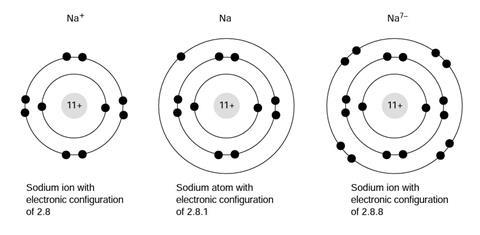1. Choose one of the four statements, and explain why you think this was the answer.
1. Na+is more stable than Na
2. Na+and Na are equally stable
3. Na+is less stable than Na
4. I do not know
5. Why do you think this was the answer?
2. Choose one of the four statements, and explain why you think this was the answer.
1. Na is more stable than Na7–
2. Na and Na7– are equally stable
3. Na is less stable than Na7–
4. I do not know
3. Choose one of the four statements, and explain why you think this was the answer.
1. Na7– is more stable than Na+
2. Na7– and Na+are equally stable
3. Na7– is less stable than Na+
4. I do not know

## Chemical stability (2)

The diagrams below represent three chemical species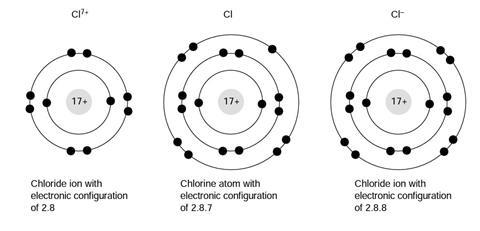1. Choose one of the four statements, and explain why you think this was the answer.
1. Cl7+ is more stable than Cl
2. Cl7+ is more stable than Cl
3. Cl7+ is less stable than Cl
4. I do not know
2. Choose one of the four statements, and explain why you think this was the answer.
1. Cl is more stable than Cl
2. Cl and Clare equally stable
3. Cl is less stable than Cl
4. I do not know
3. Choose one of the four statements, and explain why you think this was the answer.
1. Clis more stable than Cl7+
2. Cland Cl7+are equally stable
3. Clis less stable than Cl7+
4. I do not know

## Chemical stability (3)

The diagrams below represent three chemical species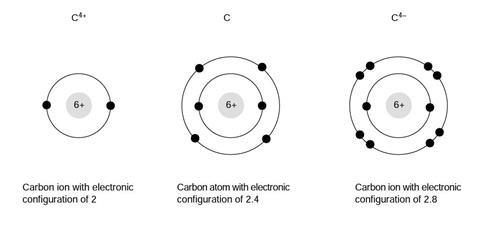1. Choose one of the four statements, and explain why you think this was the answer.
1. C4+is more stable than C
2. C4+ and C are equally stable
3. C4+ is less stable than C
4. I do not know
2. Choose one of the four statements, and explain why you think this was the answer.
1. C is more stable than C4–
2. C and C4–are equally stable
3. C is less stable than C4–
4. I do not know
3. Choose one of the four statements, and explain why you think this was the answer.
1. C4–is more stable than C4+
2. C4–and C4+are equally stable
3. C4–is less stable than C4+
4. I do not know

## Chemical stability (4)

The diagrams below represent three chemical species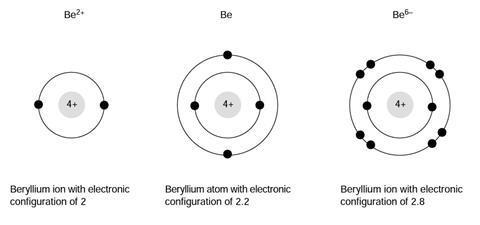1. Choose one of the four statements, and explain why you think this was the answer
1. Be2+ is more stable than Be
2. Be2+ and Be are equally stable
3. Be2+ is less stable than Be
4. I do not know
2. Choose one of the four statements, and explain why you think this was the answer
1. Be is more stable than Be6–
2. Be and Be6–are equally stable
3. Be is less stable than Be6–
4. I do not know
3. Choose one of the four statements, and explain why you think this was the answer
1. Be6– is more stable than Be2+
2. Be6– and Be2+ are equally stable
3. Be6 –is less stable than Be2+
4. I do not know

## Chemical stability (5)

The diagrams below represent three chemical species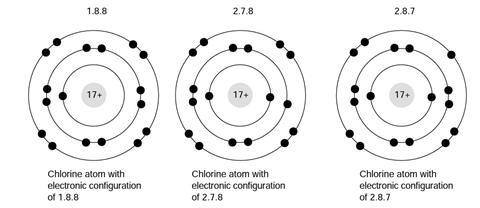1. Choose one of the four statements, and explain why you think this was the answer
1. 1.8.8 is more stable than 2.7.8
2. 1.8.8 and 2.7.8 are equally stable
3. 1.8.8 is less stable than 2.7.8
4. I do not know
2. Choose one of the four statements, and explain why you think this was the answer
1. 2.7.8 is more stable than 2.8.7
2. 2.7.8 and 2.8.7 are equally stable
3. 2.7.8 is less stable than 2.8.7
4. I do not know
3. Choose one of the four statements, and explain why you think this was the answer
1. 2.8.7 is more stable than 1.8.8
2. 2.8.7 and 1.8.8 are equally stable
3. 2.8.7 is less stable than 1.8.8
4. I do not know

## Chemical stability (6)

The diagrams below represent three chemical species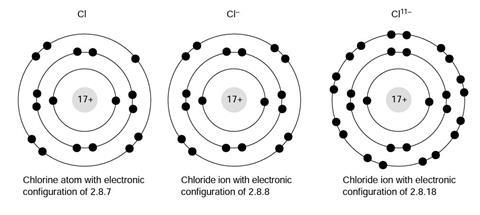1. Choose one of the four statements, and explain why you think this was the answer
1. Cl is more stable than Cl
2. Cl and Clare equally stable
3. Cl is less stable than Cl
4. I do not know
2. Choose one of the four statements, and explain why you think this was the answer
1. Clis more stable than Cl11–
2. Cland Cl11–are equally stable
3. Clis less stable than Cl11–
4. I do not know
3. Choose one of the four statements, and explain why you think this was the answer
1. Cl11– is more stable than Cl
2. Cl11– and Cl are equally stable
3. Cl11– is less stable than Cl
4. I do not know

## Chemical stability (7)

The diagrams below represent three chemical species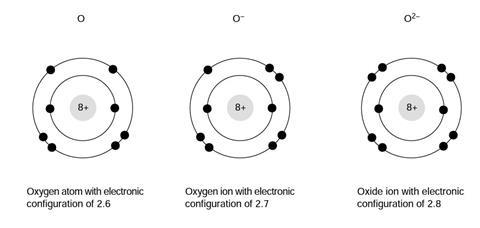1. Choose one of the four statements, and explain why you think this was the answer
1. O is more stable than O
2. O and O– are equally stable
3. O is less stable than O
4. I do not know
2. Choose one of the four statements, and explain why you think this was the answer
1. O– is more stable than O2
2. O– and O2are equally stable
3. O– is less stable than O2
4. I do not know
3. Choose one of the four statements, and explain why you think this was the answer
1. O2– is more stable than O
2. O2– and O are equally stable
3. O2– is less stable than O
4. I do not know

### Chemical stability (1)

1. The neutral atom is more stable, in the sense that the ion will spontaneously attract an electron, but the atom will not spontaneously emit an electron. Students may reasonably argue that they were thinking of real chemical contexts, where the cation is commonly found as part of real chemical substances (sodium metal, sodium chloride etc), unlike the metal. (The separate probe Stability and reactivity may be useful to follow up any ambiguity in terms of the context of the question).
2. Clearly the Na7– ion would be highly unstable, but some students will suggest it is more stable than the neutral atom due to its octet structure (full [sic] outer shell). However, in fact it is 10 electrons short of a full outer shell.
3. Clearly the Na7– ion would be highly unstable, but some students will suggest it is equally as stable as the cation due to its octet structure (full [sic] outer shell).

### Chemical stability (2)

1. A great deal of energy is required to ionise a chlorine atom to give a 7+ cation, but some students may feel the cation is more stable due to its octet structure.
2. Chlorine has an exothermic electron affinity, so the chloride ion may be considered more stable than the atom, despite the atom’s neutrality.
3. A great deal of energy is required to ionise a chlorine atom to give a 7+ cation, but some students may feel the two ions are equally stable due to their octet structures. (It is possible some may suggest that the cation is more stable, as it has a full outer shell - although many students are likely to assign full shells to both of these ions.)

### Chemical stability (3)

1. A great deal of energy is required to ionise a carbon atom to give a 4+ cation, but some students may feel the cation is more stable due to its full shell structure.
2. The C4– ion would be unstable, but some students will suggest it is more stable than the neutral atom due to its octet structure (full [sic] outer shell).
3. Both of the ions would be highly labile. Some students may feel both are stable because they have full shells, whilst others may suggest that the anion is more stable as it has an octet, or because it has more full shells.

### Chemical stability (4)

1. Energy is required to ionise the atom to form the cation, so the neutral atom should be considered more stable.
2. The highly charged anion is clearly unstable compared to the neutral atom, although some students may feel that the octet structure on the anion makes it more stable.
3. The highly charged metal anion is clearly unstable compared to the moderately charged metal cation. Some students may feel both are stable because they have full shells, whilst others may suggest that the anion is more stable as it has an octet, or because it has more full shells.

### Chemical stability (5)

1. Neither of these configurations are stable, and both would be expected to undergo spontaneous transition to 2.8.7. The 1.8.8 structure will emit a greater energy quanta as the electron drops from the n=3 to the n=1 level (cf the quantum jump from n=3 to n=2 in the 2.7.8 species). Some students may feel that both are stable as they have octets in the outer shells, and 1.8.8 may be considered more stable as it has two octets.
2. 2.8.7 is the ground state of the chlorine atom and is more stable. The excited state (2.7.8) will decay to the ground state. Some students may feel that the excited state is more stable as it has an octet in the outer shell.
3. 2.8.7 is the ground state of the chlorine atom and is more stable. The excited state (1.8.8) will decay to the ground state. Some students may feel that the excited state is more stable as it has an octet in the outer shell.

### Chemical stability (6)

1. Chlorine has an exothermic electron affinity, so the chloride ion may be considered more stable than the atom, despite the atom’s neutrality.
2. The common Cl anion is clearly more stable than the highly charged Cl11– ion. Cl has an outer octet of electrons (but not a full shell), and Cl11– has a full outer shell (but more than an octet).
3. The neutral atom is clearly more stable than the highly charged Cl11– ion. However, Cl11– has a full outer shell and may be considered more stable by some students.

### Chemical stability (7)

1. Oxygen has an exothermic electron affinity, so the O– ion may be considered more stable than the atom, despite the atom’s neutrality.
2. Although the oxide is O2– and is common, the O– anion will actually repel away another electron, so is more stable than O2. The electron affinity for the oxygen atom is -142 kJ mol–1, but the electron ‘affinity’ [sic] of the Oion is endothermic: +844 kJ mol–1.
3. The O2– ion, although common is, in isolation, less stable than the neutral atom. (The sum of the two electron affinity values is endothermic)